## The class X students school in krishnagar have been allotted a rectangular plot of land for their gardening activity. Saplings of Gulmohar are planted on the boundary at a distance of 1 m from each other. There is triangular grassy lawn in the plot as shown in the figure. The students are to sow seeds of flowering plants on the remaining area of the plot.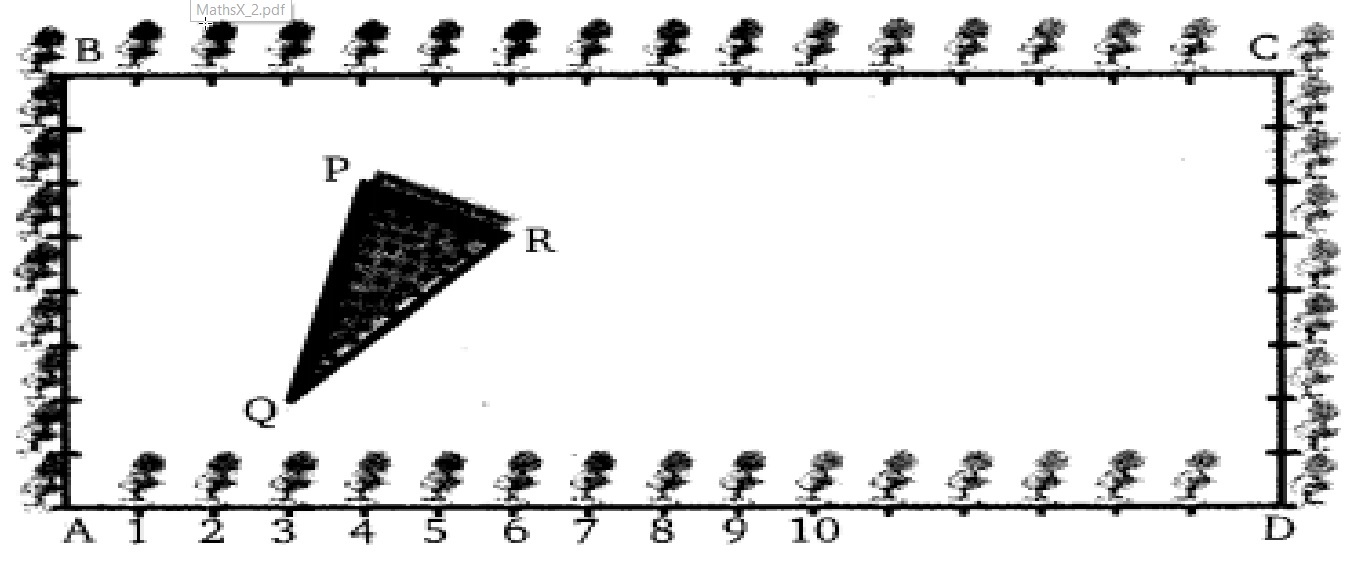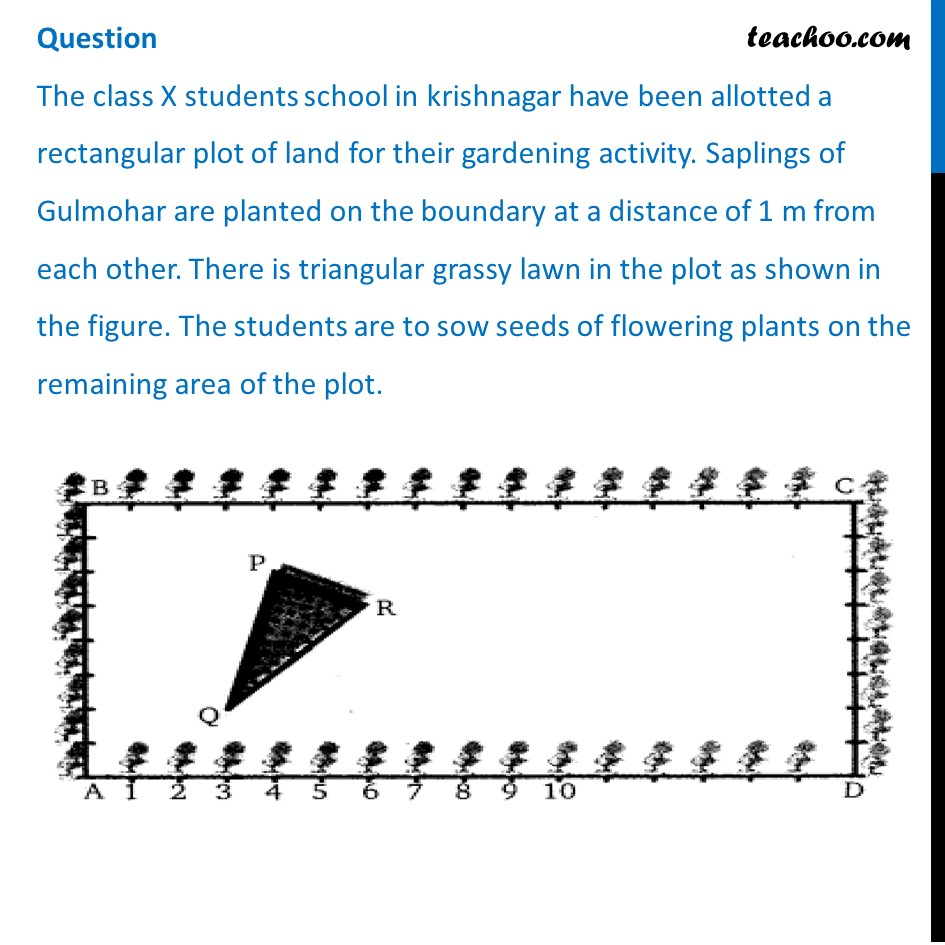## Taking A as origin, find the coordinates of P (a) (4, 6) (b) (6, 4) (c) (0, 6) (d) (4, 0)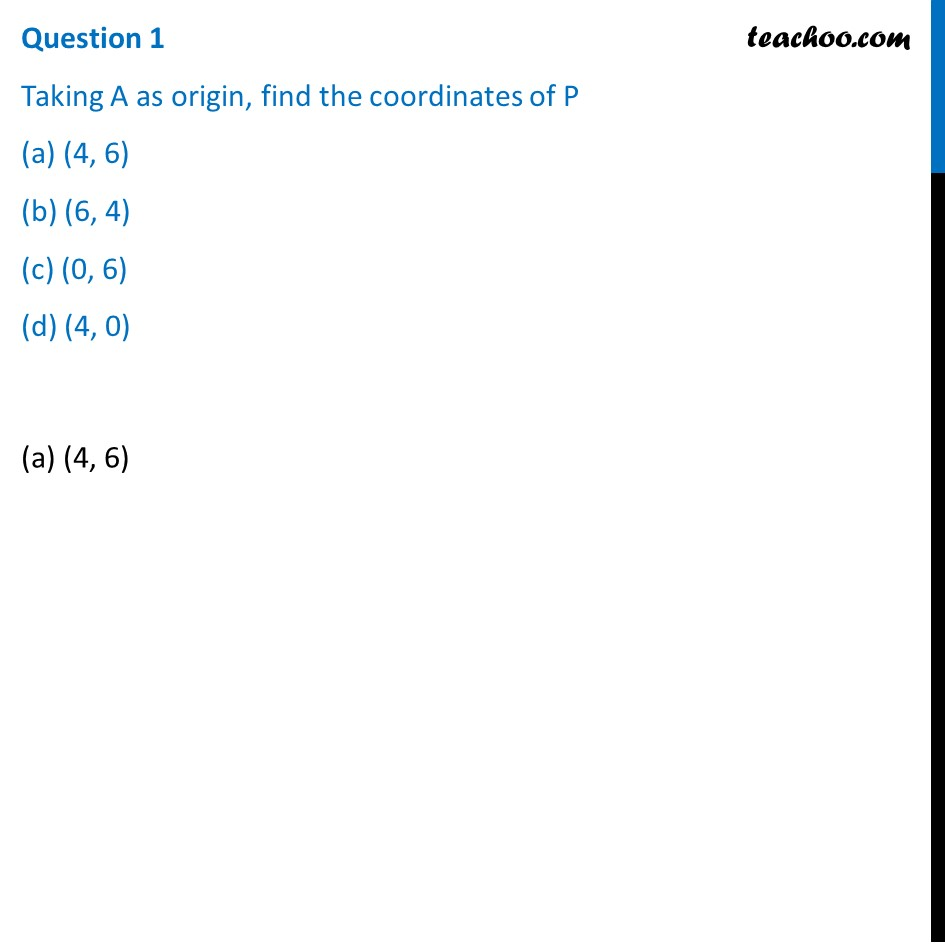## What will be the coordinates of R, if C is the origin? (a) (8, 6) (b) (3, 10) (c) (10, 3) (d) (0, 6)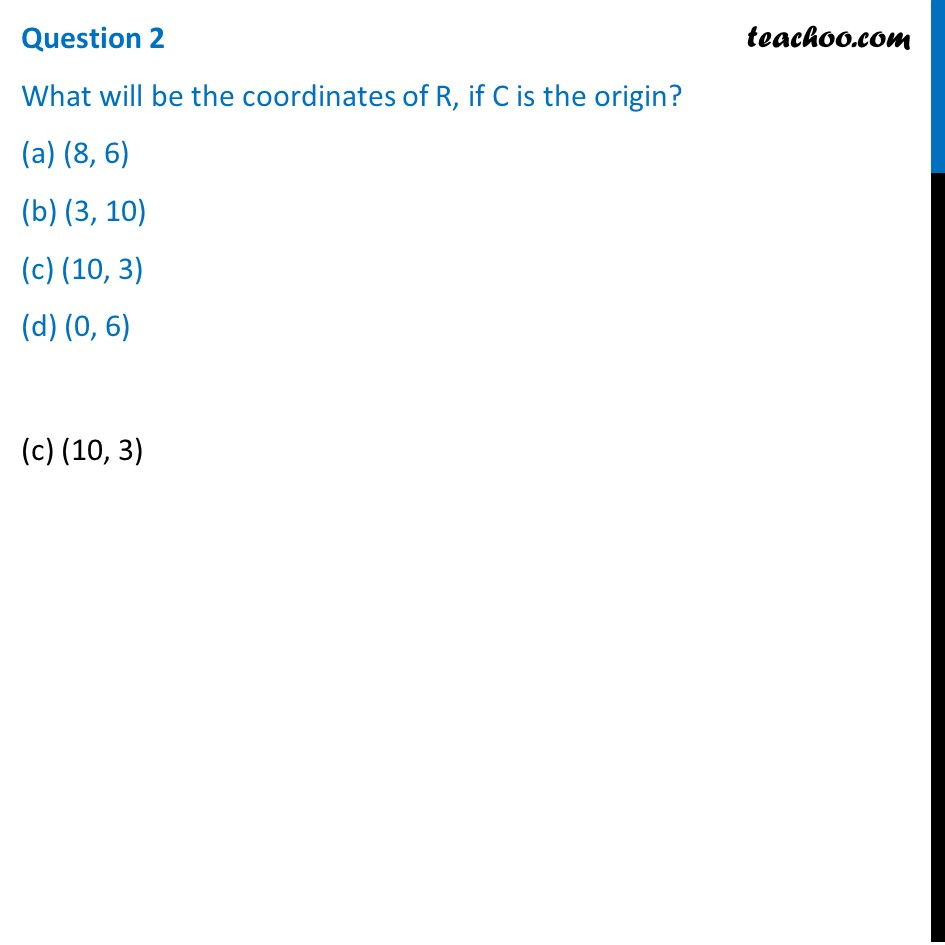## What will be the coordinates of Q, if C is the origin? (a) (6, 13) (b) (–6, 13) (c) (–13, 6) (d) (13, 6)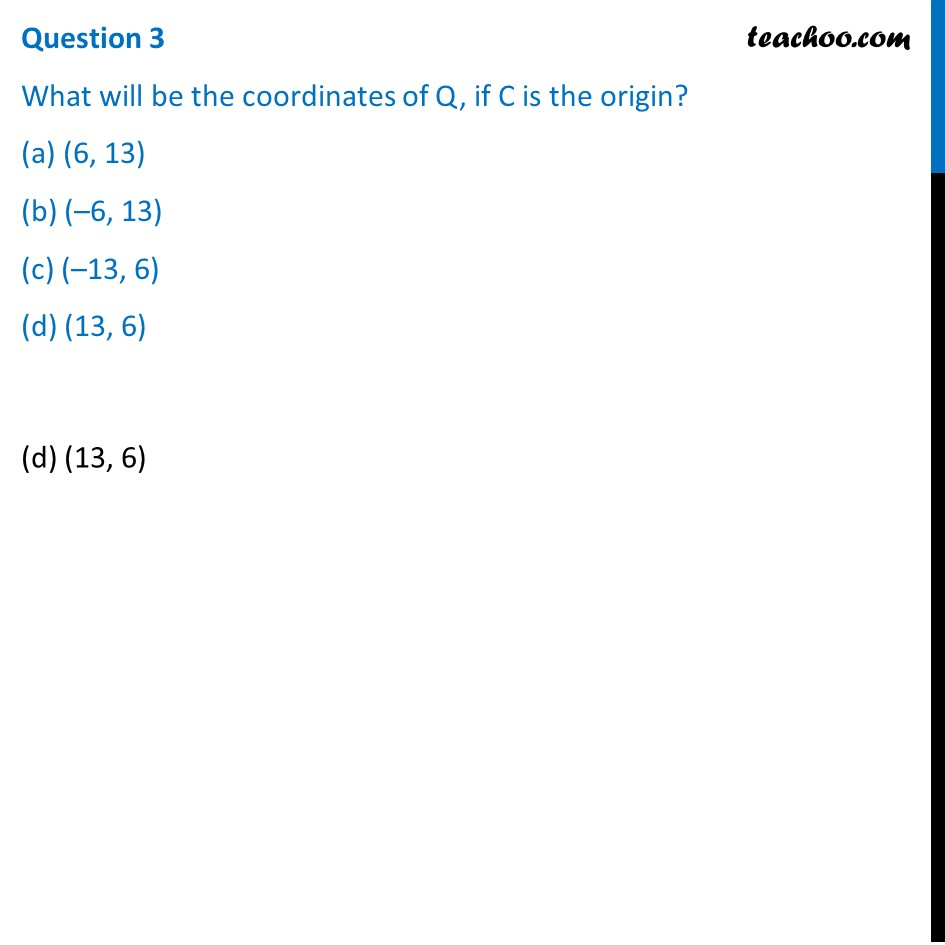## Calculate the area of the triangles if A is the origin (a) 4.5 (b) 6 (c) 8 (d) 6.25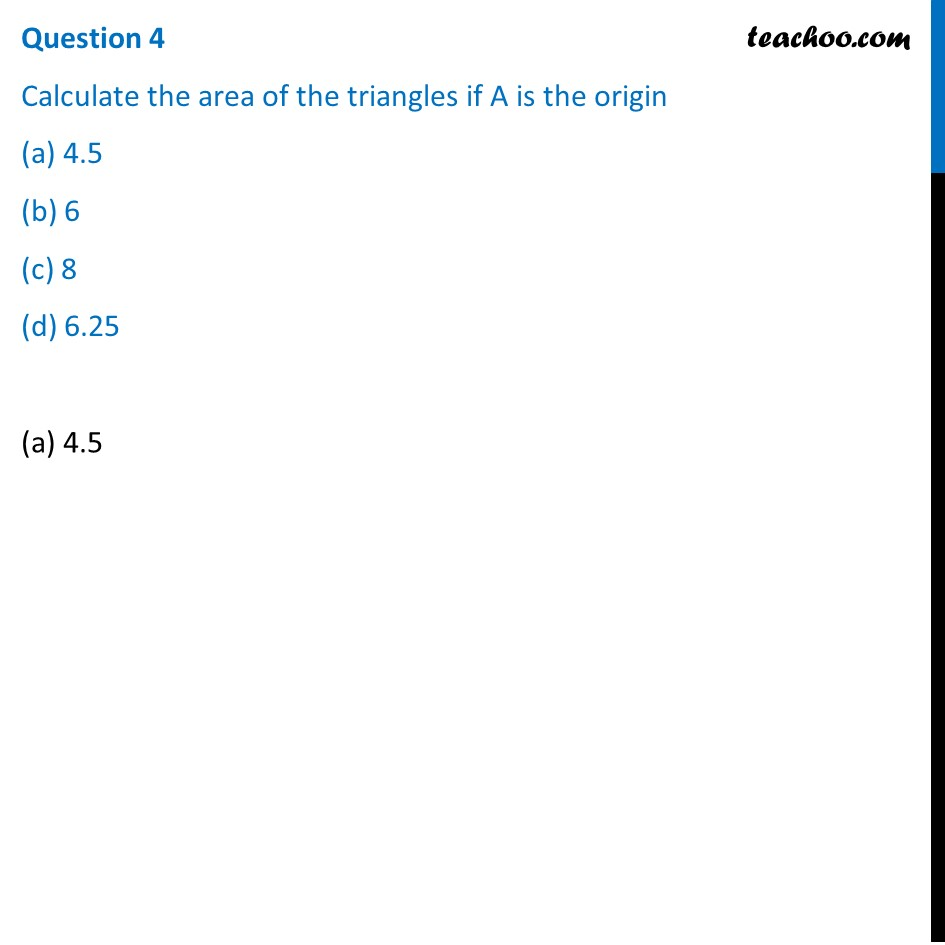## Calculate the area of the triangles if C is the origin (a) 8 (b) 5 (c) 6.25 (d) 4.5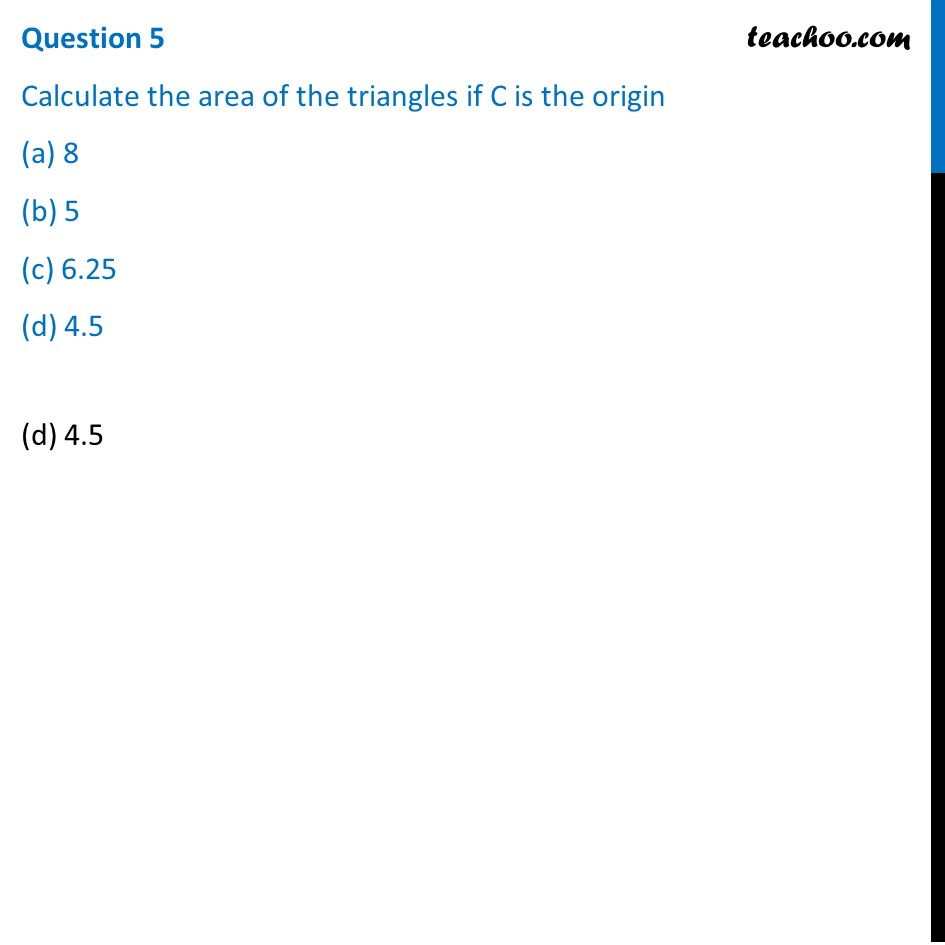1. Chapter 7 Class 10 Coordinate Geometry (Term 1)
2. Serial order wise
3. Case Based Questions (MCQ)

Transcript

Question The class X students school in krishnagar have been allotted a rectangular plot of land for their gardening activity. Saplings of Gulmohar are planted on the boundary at a distance of 1 m from each other. There is triangular grassy lawn in the plot as shown in the figure. The students are to sow seeds of flowering plants on the remaining area of the plot.Question 1 Taking A as origin, find the coordinates of P (a) (4, 6) (b) (6, 4) (c) (0, 6) (d) (4, 0)(a) (4, 6) Question 2 What will be the coordinates of R, if C is the origin? (a) (8, 6) (b) (3, 10) (c) (10, 3) (d) (0, 6)(c) (10, 3) Question 3 What will be the coordinates of Q, if C is the origin? (a) (6, 13) (b) (–6, 13) (c) (–13, 6) (d) (13, 6) (d) (13, 6) Question 4 Calculate the area of the triangles if A is the origin (a) 4.5 (b) 6 (c) 8 (d) 6.25(a) 4.5 Question 5 Calculate the area of the triangles if C is the origin (a) 8 (b) 5 (c) 6.25 (d) 4.5(d) 4.5

Case Based Questions (MCQ)